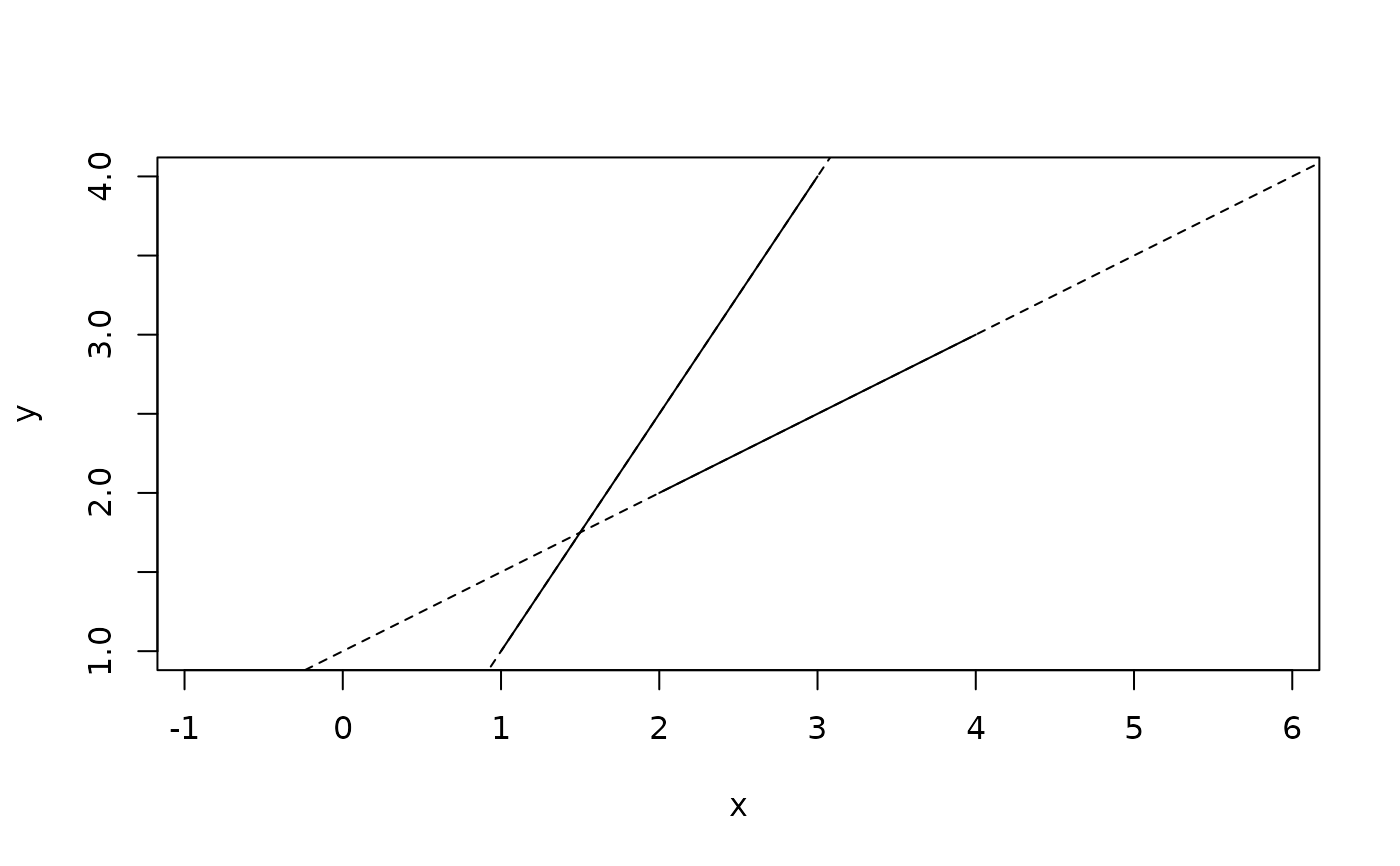A segment is a finite directed line going from one point to another. If the two points are equal it is considered to be degenerate. A segment can be flipped be taking its negative.

## Usage

segment(..., default_dim = 2)

is_segment(x)

as_segment(x)

## Arguments

...

Various input. See the Constructor section.

default_dim

The dimensionality when constructing an empty vector

x

A vector of segments or an object to convert to it

## Value

An euclid_segment vector

## Constructors

2 and 3 dimensional segments

• Providing two points will construct segments starting at the first point and ending at the second

• Providing a point and a vector will construct segments starting at the point and ending at the point defined by the start point plus the vector

Other Geometries: circle(), direction(), iso_cube(), iso_rect(), line(), plane(), point(), ray(), sphere(), tetrahedron(), triangle(), vec(), weighted_point()

Other Curves: line(), ray()

## Examples

# Construction
p <- point(sample(4), sample(4))
s <- segment(p[1:2], p[3:4])
s
#> <2D segments >
#>  [<x:3, y:4>, <x:1, y:1>] [<x:2, y:2>, <x:4, y:3>]

plot(s)

segment(p[1:2], as_vec(p[3:4]))
#> <2D segments >
#>  [<x:3, y:4>, <x:4, y:5>] [<x:2, y:2>, <x:6, y:5>]

# Flip segments
-s
#> <2D segments >
#>  [<x:1, y:1>, <x:3, y:4>] [<x:4, y:3>, <x:2, y:2>]

# Segments can be converted to vectors, directions and lines
as_vec(s)
#> <2D vectors >
#>  <x:-2, y:-3> <x:2, y:1>

as_direction(s)
#> <2D directions >
#>  <dx:-2, dy:-3> <dx:2, dy:1>

as_line(s)
#> <2D lines >
#>  <a:3, b:-2, c:-1> <a:-1, b:2, c:-2>
plot(s)
euclid_plot(as_line(s), lty = 2)